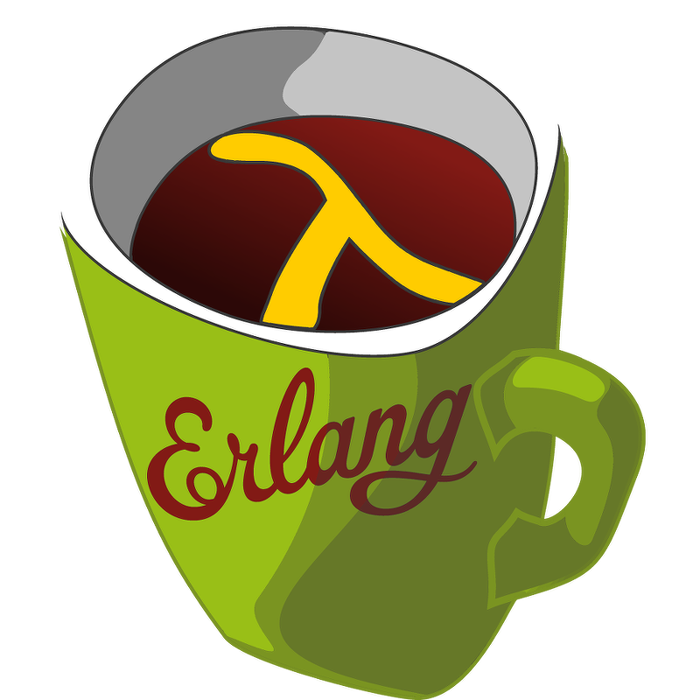This week's LFE Friday was translated with permission from the Erlang Thursday series by Steven Proctor. This week's translator: Robert Virding.

Today's LFE Friday is on dict:merge/3.

`dict:merge/3` takes 3 arguments, the first argument is a merge function to be called when there is a key collision, and the second and third arguments are dictionaries.

The merge function is a function that takes the key as the first argument, the value from the first dictionary as the second argument, and the value from the second dictionary as the the third argument.

``````> (dict:to_list
(dict:merge (lambda (k value1 value2) (list value1 value2))
(dict:from_list '(#(a 1) #(b 2) #(x 5)))
(dict:from_list '(#(x 7) #(y 8) #(z 10)))))
(#(a 1) #(b 2) #(x (5 7)) #(y 8) #(z 10))
> (dict:to_list
(dict:merge (lambda (k value1 value2) (* value1 value2))
(dict:from_list '(#(a 1) #(b 2) #(x 5)))
(dict:from_list '(#(x 7) #(y 8) #(z 10)))))
(#(a 1) #(b 2) #(x 35) #(y 8) #(z 10))``````

The merge function passed to `dict:merge/3` only gets called in the case of a collision, as shown below. Note that there is a call to `exit/1` in the body of the function which would cause the process to terminate if the function was ever invoked.

``````> (dict:to_list
(dict:merge (lambda (k value1 value2) (exit 'merge-happened))
(dict:from_list '(#(a 1) #(b 2)))
(dict:from_list '(#(x 7) #(y 8) #(z 10)))))
(#(a 1) #(b 2) #(x 7) #(y 8) #(z 10))``````

If you wish to treat the merge as an overlay of the second dictionary over the first, the merge function just needs to return the value from the second dictionary in the case of a key conflict.

``````> (dict:to_list
(dict:merge (lambda (k value1 value2) value2)
(dict:from_list '(#(a 1) #(b 2) #(x 5)))
(dict:from_list '(#(x 7) #(y 8) #(z 10)))))
(#(a 1) #(b 2) #(x 7) #(y 8) #(z 10))``````

If you want to keep all of the keys and values in the first dictionary, and just add the keys and values that are in the second dictionary, but not in the first dictionary, the merge function should just return the value associated with the first dictionary.

``````> (dict:to_list
(dict:merge (lambda (k value1 value2) value1)
(dict:from_list '(#(a 1) #(b 2) #(x 5)))
(dict:from_list '(#(x 7) #(y 8) #(z 10)))))
(#(a 1) #(b 2) #(x 5) #(y 8) #(z 10))``````

Just a peek into the new Maps that came in to Erlang in the 17.0 release.

-Proctor, Robert

01 March 2015

tutorials